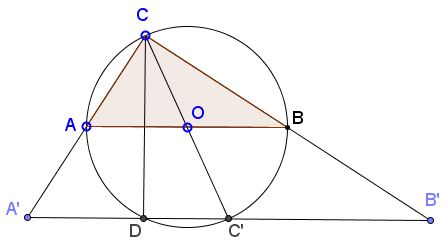# Pythagoras, from the Power of a Point - Again

Several times previously (proofs 22, 43, 71) the Pythagorean theorem has been derived from the Power of a Point theorem. Below is another example of the power of that theorem devised by Bùi Quang Tuån. Bùi's approach is illustrated by the following diagramLet $ABC$ be a right triangle $(\angle ACB=90^{\circ}),$ with the sides $a=BC,\,b=AC,\,c=AB,$ as usual. We are to prove that $a^{2}+b^{2}=c^{2}.$

Let $A'$ be the reflection of $C$ in $A,$ $B'$ in $B,$ $C'$ in $O$ the circumcenter of $\Delta ABC$ (the midpoint of $AB).$ The three points $A',\,B'\,C'$ are obtained by a homothety with center $C$ and coefficient $2$ from $A,\,O,\,B$ and, therefore, are collinear. Line $A'B'$ meets the circumcircle $(ABC)$ in $C'$ and the second time in $D.$

Note that $A'C=2b,\,B'C=2a,\,A'B'=2c,$ whereas $A'C'=B'C'=c.$ Assuming, as depicted in the diagram, that $a\gt b,$ we have by the Power of a Point theorem,

• $A'A\cdot A'C=A'D\cdot A'C',$ i.e., $2a^{2}=(c-DC')\cdot c,$
• $B'B\cdot B'C=B'D\cdot B'C',$ i.e., $2b^{2}=(c+DC')\cdot c.$

Adding the two gives $2a^{2}+2b^{2}=2c^{2}$ - one step away from the Pythagorean theorem.